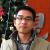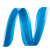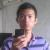## javascript中正则诡异行为求解!

scl33 发布于 2011/09/06 13:16

```var ptn = /^\d+\$/gi;//很简单的全数字判断, 由ptn引用, 以后经常重复使用
ptn.test('123');//true
ptn.test('123');//false!
ptn.test('123');//true
ptn.test('123');//false!
/^\d+\$/gi.test('123');//true
/^\d+\$/gi.test('123');//true
/^\d+\$/gi.test('123');//true```

P.S. 开始我认为会不会是浏览器支持的差异, 测试或CHROME 13和IE 9都一样..无语了..

0#### 引用来自“phpcake”的答案

ptn引用了一个正则对象，

The object associated with this property is always the global RegExp object.

The lastIndex property is zero-based, that is, the index of the first character is zero. Its initial value is –1. Its value is modified whenever a successful match is made.

The lastIndex property is modified by the exec and test methods of the RegExp object, and the matchreplace, and split methods of the String object.

The following rules apply to values of lastIndex:

• If there is no match, lastIndex is set to -1.

• If lastIndex is greater than the length of the string, test and exec fail and lastIndex is set to -1.

• If lastIndex is equal to the length of the string, the regular expression matches if the pattern matches the empty string. Otherwise, the match fails and lastIndex is reset to -1.

• Otherwise, lastIndex is set to the next position following the most recent match.

还得补充一点, 在使用test()方法时, 只有开启了全局属性时(/g), 才会使用并更新lastIndex, 否则每次都从0开始搜索.

另外, 经测试, 搜索不到时, lastIndex被重置为0, 而不是-1.

最后, 关于为何编译后即正常使用的问题, 经测试, 在编译后, 自动修正了g选项所致, 因为已定义^和\$(从开头到结尾), 我们可以看到编译前global属性为true, 编译后变成了false.0/^\d+\$/gi 这里面 这个g 去掉之后 都是 true 了0ptn引用了一个正则对象，0015.10.6.2 R egExp.prototype . exe c ( s t r ing)
Performs a regular expression match of string against the regular expression and returns an Array
object containing the results of the match, or null if the string did not match
The string ToString(string) is searched for an occurrence of the regular expression pattern as follows:
1. Let S be the value of ToString(string).
2. Let length be the length of S.
3. Let lastIndex be the value of the lastIndex property.
4. Let i be the value of ToInteger(lastIndex).
5. If the global property is false, let i = 0.
6. If I < 0 or I > length then set lastIndex to 0 and return null.
7. Call [[Match]], giving it the arguments S and i. If [[Match]] returned failure, go to step 8;
otherwise let r be its State result and go to step 10.
8. Let i = i+1.
9. Go to step 6.
10. Let e be r's endIndex value.-  1 4 5  -
11. If the global property is true, set lastIndex to e.
12. Let n be the length of r's captures array. (This is the same value as 15.10.2.1's
NCapturingParens.)
13. Return a new array with the following properties:
•  The index property is set to the position of the matched substring within the complete string
S.
•  The input property is set to S.
•  The length property is set to n + 1.
•  The 0 property is set to the matched substring (i.e. the portion of S between offset i inclusive
and offset e exclusive).
•  For each integer i such that I > 0 and I ≤ n, set the property named ToString(i) to the i
th
element
of r's captures array.